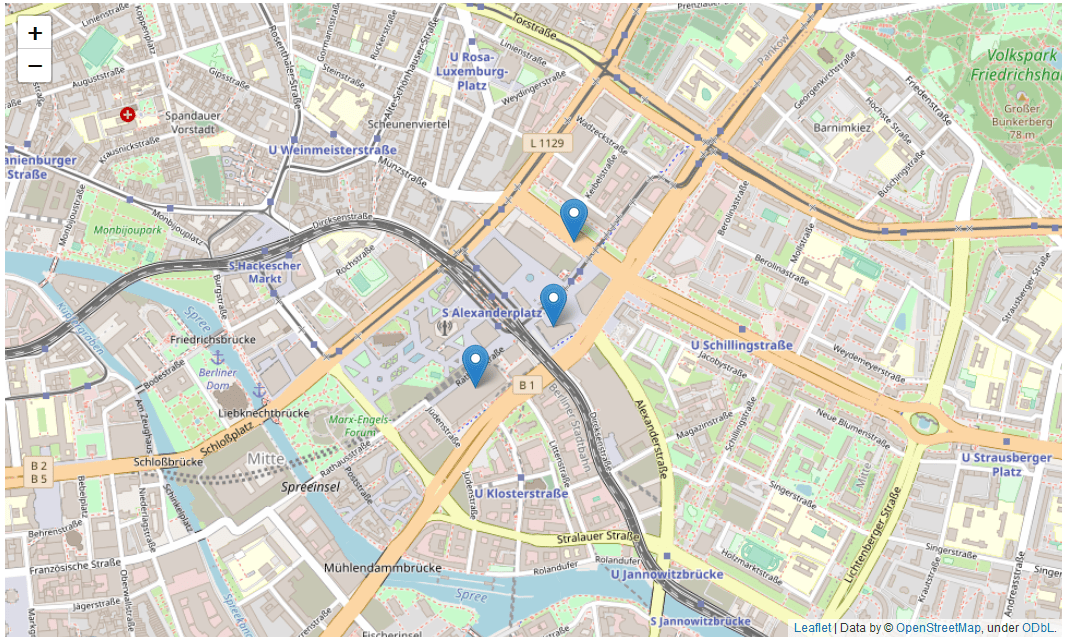# Multiple geocoded map markers with Geopy and Folium in PythonIn a previous post I have already demonstrated how to use Nominatim in Python (using the Geopy module) to geocode a location name into longitude and latitude coordinates.

In this post I want to show how one can geocode a list of locations using Geopy.

For this I will start by using the Pandas module for reading in a simple and brief csv-file containing location names as specified by entries in a country, city and street column:

```# importing pandas
import pandas
# read in csv file containing location data
# display table from csv file
data
```

Let’s check the datatype of the tabular data:

```type(data)
```
`pandas.core.frame.DataFrame`

Now that I have read in the data I will geocode locations and assign geocoded coordiantes to a new column. Since the data is a pandas DataFrame I can make use of the apply() methode to apply the relevant Nominatim geocoding service to every address in the dataframe.

First I need to converge all column entries into addresses, adding those into a new column in the tabular DataFrame. Then, I can create a service object referencing the Nominatim Geopy service and apply that service to every location, returning the geocoded result into an additional new column:

```# merging country, city and street into a single address string
data["addresses"] = data["country"] + ", " + data["city"] + ", " + data["street "]
# import the geopy module
import geopy
# create a service object
service = geopy.Nominatim(user_agent = "myGeocoder")
# geocode every address, using .apply() methode for pandas DataFrame
from geopy.extra.rate_limiter import RateLimiter
# display tabular data
data
```

Let us check the data type of the “coodinate” column entries:

```type(data["coordinates"])
```
`geopy.location.Location`

The geocoded locations are of type Geopy Location. Objects of the Location class posses various attributes. One of them being latitude and another one being longitude:

```data["coordinates"].longitude
```
`13.4144809`
```data["coordinates"].latitude
```
`52.5228654`

I now calculate the “mean” longitude and latitude scores. I want to use them as center point of my Folium location marker map plot:

```# extracting longitude and latitude values to separate lists
longs = [coord.longitude for coord in data["coordinates"]]
lats = [coord.latitude for coord in data["coordinates"]]
# calculating mean longitude and latitude values
import statistics
meanLong = statistics.mean(longs)
meanLat = statistics.mean(lats)
# display result
print("meanLong = " + str(meanLong) + "; meanLat = " + str(meanLat))
```
```meanLong = 13.412910038576356; meanLat = 52.52100943333333
```
```[longs,lats]
```
```[[13.4144809, 13.4136431, 13.410606115729072],
[52.5228654, 52.5208149, 52.519348]]```

Using the Folium module I can now create markers for the locations and plot them on map tiles:

```# import folium
import folium
# create a base map centered around Berlin
mapObj = folium.Map(location = [meanLat,meanLong], zoom_start = 15)
# create marker object for Berlin, one by one for every location in data DataFrame
for i in range(0,data.shape): # .shape for Pandas DataFrame is the number of rows
# create marker for location i
markerObj = folium.Marker(location = [lats[i],longs[i]])